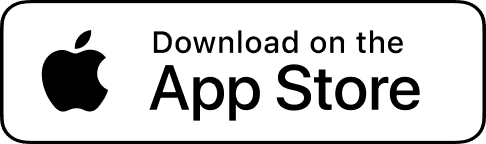Answer.AIHELPPPPPPPP! Which is true regarding secants and chords?

Chords intersect the perimeter of a circle at two points. Secants intersect the perimeter of a circle once.
Chords intersect the perimeter of a circle at one point. Secants intersect the perimeter of a circle twice.
Chords and secants intersect the perimeter of a circle twice. Chords are segments entirely within a circle, while secants are lines or rays that extend through a circle.
Chords and secants intersect the perimeter of a circle twice. Chords are lines or rays that extend through a circle, while secants are segments entirely within a circle.Solve problem with AIChords and secants intersect the perimeter of a circle twice. Chords are segments entirely within a circle, while secants are lines or rays that extend through a circle.

Explanation:

A line intersecting a circle in two places is referred to as a secant.  The portion of the secant contained within the circle is called a chord.

This means that both chords and secants intersect the perimeter of a circle twice, chords are entirely inside the circle, and secants extend through a circle to the outside.

Recommend videos
You might be interested in...
Explore more...